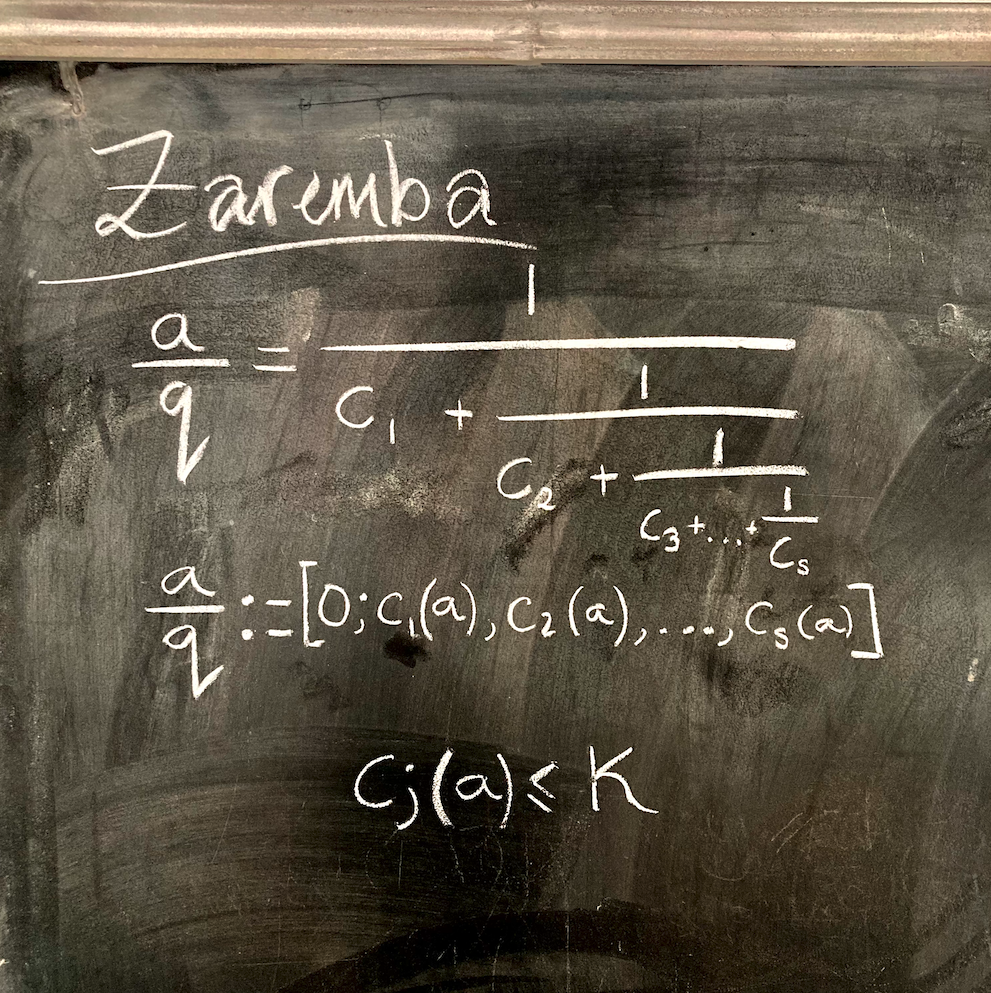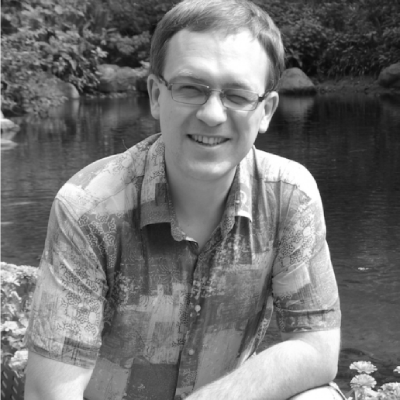# A new bound concerning Zaremba’s conjecture

## 5 pm, 29 Mar 2023

Prof. Ilya Shkredov discusses Zaremba’s elegant 1971 conjecture in the theory of continued fractions, and explores the bounds relating to it.

By the Euclidean algorithm, any rational number $a/q$ can be uniquely represented as a regular continued fraction. In the theory of continued fractions, in 1971 Zaremba famously made the following conjecture: there is an absolute constant $k$ such that for any positive integer $q$, there exists $a$, which is coprime to $q$, such that in the continued fraction expansion of $a/q$, all partial quotients are less than or equal to $k$. Zaremba himself conjectured that the value of this absolute constant $k$ equals 5. Two decades later, Hensley conjectured that for a large, prime denominator, a bound equal to 2 is sufficient. Ever since, further tightening this bound has been an ongoing problem in the number theory community.

In this talk and drinks, Prof. Ilya Shkredov presents a marked improvement to the well-known Korobov bound on this conjecture. In 1963, using exponential sums, Korobov proved that for any prime $q$, there exists a coprime numerator such that the partial quotients never exceed log($q$). Shkredov presents a proof that, for any sufficiently large prime $q$, the partial quotients are bounded by $O$(log $q$/ log log $q$).

## Event info

This event is at 5pm on Wednesday, 29 March, on the second floor of the Royal Institution. Grab a drink and say hi before the talk starts at 5:15 in Tyndall’s Parlour. After the talk, everyone meets for discussion over drinks in the Old Post Room. To attend, email at@lims.ac.uk.## SpeakersProf. Ilya Shkredov is an Arnold Fellow at the London Institute. He studied mathematics at Moscow State University, IAS Princeton and MSRI Berkley. His work includes generalisations of Szemerédi's theorem, the sum-product phenomenon, and inverse results in additive combinatorics.